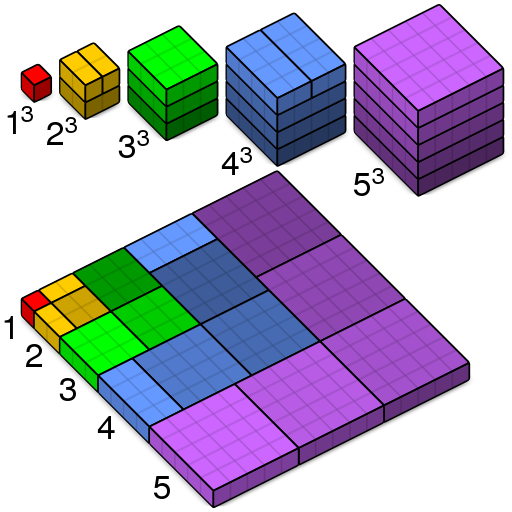# Cubes and SquaresImage: Wikimedia Commons

MATLAB’s Loren Shure devised this lovely “proof without words” of Nicomachus’ theorem, that the sum of the first n cubes is the square of the nth triangular number:$\displaystyle 1^{3}+2^{3}+3^{3}+\cdots +n^{3}=\left(1+2+3+\cdots +n\right)^{2},$

R.J. Stroeker of Erasmus University wrote, “Every beginning student of number theory surely must have marveled at [this] miraculous fact.”